#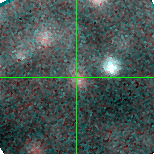PSO-J10.1165+40.7082 (J004027.99+404229.0)

## Target Notes### Classification: -

Unresolved from LGGS J004027.98+404229.5 of comparable brightness 0.5 arcseconds away. LGGS J004028.07+404231.1 of comparable brightness 2.2 arcseconds NE.

## Plots of Brightness and Color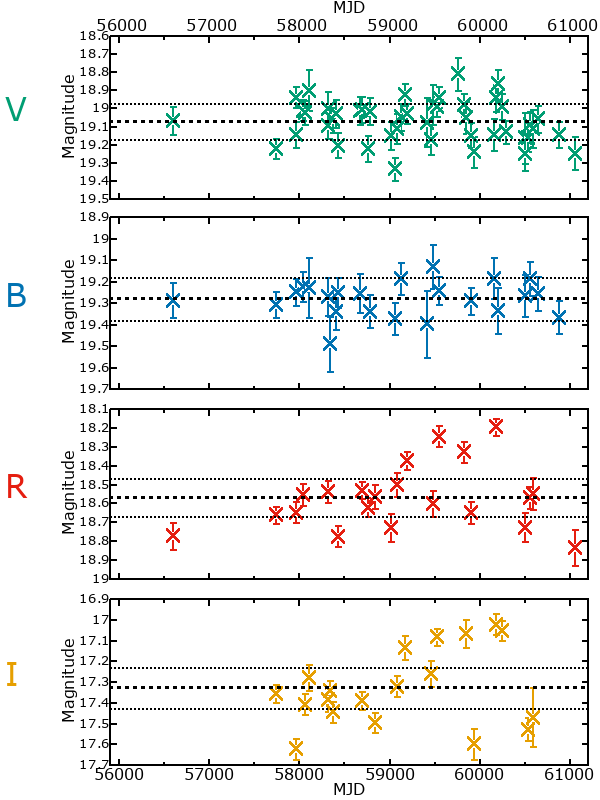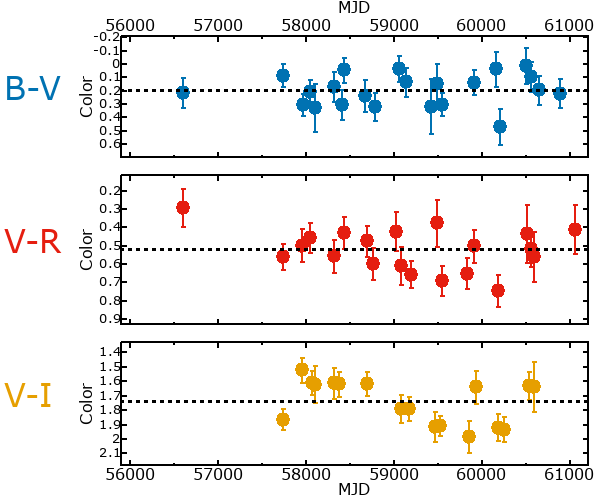## Variability

From the data available in this survey, it is not possible to tell with certainty if this star is variable.

### Correlation factors between bands

If the brightness variation is true variability then there should be a correlation between brightness changes recorded in different filter bands. The following table are the R-squared linear correlation factors calculated for the pairs of bands. The number in parentheses next to the R-squared value is the number of elements used to calculate the correlation factor. The number in square brackets next to the band is the range of magnitudes measured.

V [0.30 mag]B [0.34 mag]R [0.41 mag]
B [0.30 mag]0.01 (10)
R [0.41 mag]0.27 (11)0.31 (5)
I [0.51 mag]0.45 (9)0.21 (3)0.48 (5)

### Magnitude-Magnitude Plots

The plots below are provided to check the values in the table above and also to show any non-linear correlations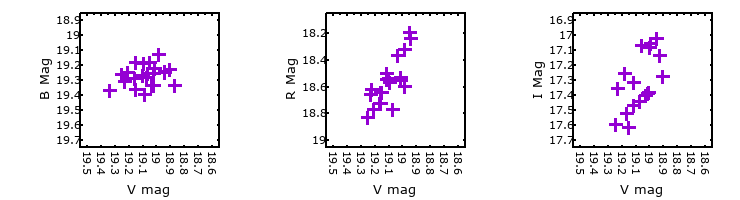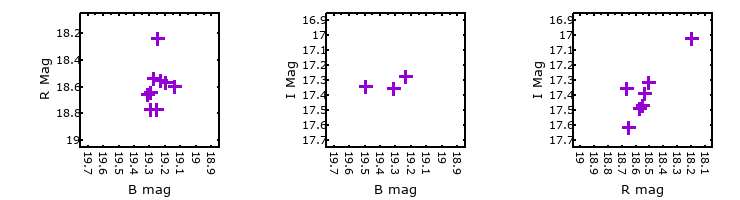### I & R Statistics

Welch & Stetson (1993, AJ, 105, 1813) present a method for varible star detection in CCD images from automated surveys. Data from two bands are compared and two statistics are calculated: R and I.

The I-statistic has an expectation value of zero for stars that are NOT variable. A larger non-zero I-value indicates a higher likelihood that the star is variable. The R statistic indicates how the color of the star changes as the variable grows brighter with R = 1.0 being no change in color.

R and I values have only been calculated if there are more than four (4) matched measurements of brightness in each band.
V
(I|R)
B
(I|R)
R
(I|R)
B
(I|R)
-0.06 | 0.59
R
(I|R)
1.84 | 0.610.46 | 0.05
I
(I|R)
2.47 | 0.80--- | --- 2.35 | 0.62